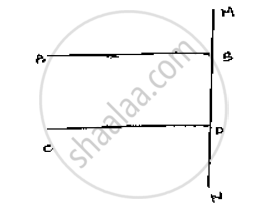# Prove that the Straight Lines Perpendicular to the Same Straight Line Are Parallel to One Another. - Mathematics

Prove that the straight lines perpendicular to the same straight line are parallel to one
another.

#### Solution

Let  AB and CD perpendicular to the Line MN∠ABD = 90°            [∵ AB  ⊥ MN ]     ......(i )

∠CON = 90°          [ ∵ CD ⊥ MN]      ......(ii)

Now,

∠ABD = ∠CDN = 90°                    [From (i) and (ii)]

AB || CD, Since corresponding angles are equal.

Concept: Parallel Lines and a Transversal
Is there an error in this question or solution?

#### APPEARS IN

RD Sharma Mathematics for Class 9
Chapter 10 Lines and Angles
Exercise 10.4 | Q 11 | Page 47